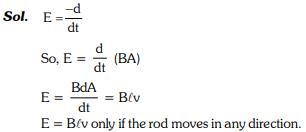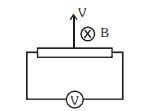Courses

# Test: Electromagnetic Induction Level- 1

## 30 Questions MCQ Test Physics Class 12 | Test: Electromagnetic Induction Level- 1

Description
This mock test of Test: Electromagnetic Induction Level- 1 for Class 12 helps you for every Class 12 entrance exam. This contains 30 Multiple Choice Questions for Class 12 Test: Electromagnetic Induction Level- 1 (mcq) to study with solutions a complete question bank. The solved questions answers in this Test: Electromagnetic Induction Level- 1 quiz give you a good mix of easy questions and tough questions. Class 12 students definitely take this Test: Electromagnetic Induction Level- 1 exercise for a better result in the exam. You can find other Test: Electromagnetic Induction Level- 1 extra questions, long questions & short questions for Class 12 on EduRev as well by searching above.
QUESTION: 1

### electromagnetic induction i.e currents can be induced in coils (Select the best)

Solution:

Explanation:for electromagnetic induction magnet and coil both may move but relative motion between must be present

QUESTION: 2

### Damping in galvanometers is based oUpdate Questionn

Solution:

Explanation : The coil of galvanometer is wound on a light metal frame. When the coil and frame rotate in the field of the permanent magnet, the eddy current set up in the frame oppose the motion so that the coil returns to zero quickly.

QUESTION: 3

### Predict the polarity of the capacitor in the situation described by fig.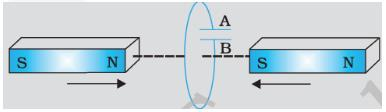Solution:

Explanation:A will become positive with respect to B because current  indused is in clockwise direction

QUESTION: 4

A circular coil of radius 8.0 cm and 20 turns is rotated about its vertical diameter with an angular speed of 50 rad s-1 in a uniform horizontal magnetic field of magnitude 3×10-2 T. If the coil resistance is 10Ω, maximum emf induced in the coil,maximum value of current in the coil and average power loss due to Joule heating are

Solution:

e = NBAω = 20 x 3 x 10-2 x (3.14 x 0.08 x 0.08) x 50 = 0.603C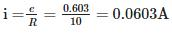average power loss due to joule heating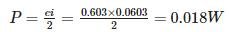QUESTION: 5

An ac generator consists of 8 turns of wire, each of area A=0.0900m2 , and the total resistance of the wire is 12.0Ω. The loop rotates in a 0.500-T magnetic field at a constant frequency of 60.0 Hz. Maximum induced emf is

Solution:

e = NBAω = NBA2πn = 8 x 0.5 x 0.09 x 2 x 3.14 x 60 = 136V

QUESTION: 6

When a bar magnet is pushed towards the coil connected in series with a galvanometer, the pointer in the galvanometer G

Solution:

Explanation:When  bar is in motion then flux will changed hence current will be induced.

QUESTION: 7

There are two stationary coils near each other. If current in coil-1 is changing with time, relationship of current in coil-2 to it's emf is described by

Solution: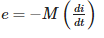M is mutual inductance

QUESTION: 8

A metallic rod of 1 m length is rotated with a frequency of 50 rev/s about an axis passing through the centre point O. Other end of the metallic rod slides on a Metallic ring . A constant and uniform magnetic field of 2 T parallel to the axis is present everywhere. What is the emf between the centre and the metallic ring?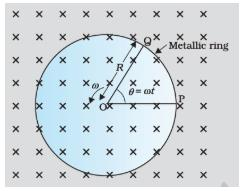Solution: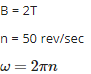l = 1 m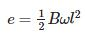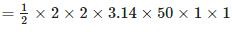= 314V

QUESTION: 9

Coils C1 and C2 are stationary. C1 connected in series with a galvanometer while C2 is connected to a battery through a tapping key. If the tapping key is pressed and released the galvaometer

Solution:

Explanation:flux will be changed when current in C2 is changing. Hence current will be induced in coil C1.

QUESTION: 10

An AC generator consists of

Solution:

Explanation:AC generators  operate on the same fundamental principles of electromagnetic induction.The turning of a coil in a magnetic field produces motional emfs in both sides of the coil.A slip ring  is a method of making an electrical connection through a rotating assembly. Formally, it is an electric transmission device that allows energy flow between two electrical rotating parts, such as in a motor.

QUESTION: 11

Solution:

Explanation:The induced electromotive force in any closed circuit is equal to the negative of the time rate of change of the magnetic flux enclosed by the circuit.

QUESTION: 12

Current in a circuit falls from 5.0 A to 0.0 A in 0.1 s. If an average emf of 200 V induced, estimate of the self-inductance of the circuit is

Solution: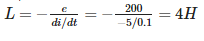QUESTION: 13

The magnetic field between the Horizontal poles of an electromagnet is uniform at any time, but its magnitude is increasing at the rate of 0.020T/s.The area of a horizontal conducting loop in the magnetic field is 120cm2, and the total circuit resistance, including the meter, is 5Ω. Induced emf and the induced current in the circuit are

Solution:

Explanation:

e = Rate of change of magnetic field x area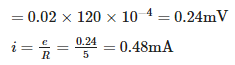QUESTION: 14

Coils C1 and C2 are stationary. C1 connected in series with one very small diwali bulb while C2 is connected to a battery .If the connection is suddenly snapped

Solution:

Explanation:Flux will be changed due to this current is induced momentarily

QUESTION: 15

If AC generator coil has area A, rotates in a magnetic field of B with an angular speed ω , the voltage generated is

Solution:

Explanation:e=NBAωSinωt

QUESTION: 16

Kamla peddles a stationary bicycle the pedals of the bicycle are attached to a 100 turn coil of area 0.10m2. The coil rotates at half a revolution per second and it is placed in a uniform magnetic field of 0.02 T perpendicular to the axis of rotation of the coil. What is the maximum voltage generated in the coil?

Solution:

Maximum voltage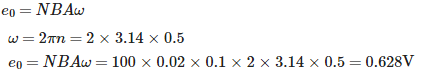QUESTION: 17

A pair of adjacent coils has a mutual inductance of 1.5 H. If the current in one coil changes from 0 to 20 A in 0.5 s, change of flux linkage with the other coil is

Solution: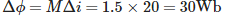QUESTION: 18

The magnetic field between the Horizontal poles of an electromagnet in is uniform at any time, but its magnitude is increasing at the rate of 0.020 T/s.The area of a loop made of perfect insulating material is 120cm2.This loop is kept horizontally in the magnetic field. Induced emf and the induced current in the circuit are

Solution:

e = Rate of change of magnetic field x area

=0.02×120×10−4=0.24mV

QUESTION: 19

If the polarity of induced emf is such that it tends to produce a current which aids the change in magnetic flux that produced it, which conservation law is violated?

Solution:

Explanation : In this case we would be obtaining electrical energy continuously without doing any work.

QUESTION: 20

A rectangular loop and a circular loop are moving out of a uniform magnetic field region to a field-free region with a constant velocity v as shown in fig. In which loop do you except  the induced EMF to be constant during the passage out of the field region if the field is normal to the loops.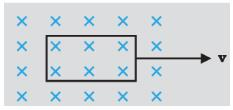Solution:

Explanation:The induced EMF is expected to be constant in the case of a rectangular loop. In case of a circular loop, the rate of change of area of the loop during the passage out of the field region is not constant, whereas it is constant for rectangular loop.

QUESTION: 21

A rectangular conductor PQRS in which the conductor PQ is free to move is kept in a magnetic field B ,which is perpendicular to the PQRS which lies in a single plane. If the conductor PQ has a length l,moves with a speed v, then induced emf is

Solution: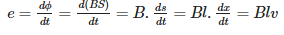QUESTION: 22

A 500-loop circular wire coil with radius 4.00 cm is placed between the poles of a large electromagnet. The magnetic field is uniform and makes an angle of 60 with the plane of the coil; it decreases at 0.200 T/s . Magnitude of induced emf is

Solution: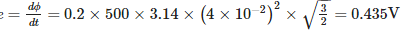QUESTION: 23

Use Lenz’s law to determine the direction of induced current in the situations described by the Figure (a) A wire of irregular shape turning into a circular shape; (b) A circular loop being deformed into a narrow straight wire. The directions for (a) and (b) respectively are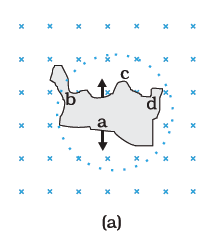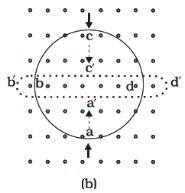Solution:

Explanation:(a) for a given Periphery the area of a circle is maximum. When a coil takes a circular shape, the magnetic flux linked with the coil increases, so the current induced in the coil will tend to decrease the flux and so it will produce a magnetic field upward. As a result the current induced in the coil will flow in anticlockwise direction.(b) When circular coil takes the shape of narrow straight wire, The magnetic flux linked with the coil decreases. So the current induced in the coil will tend to oppose the decrease in magnetic flux. Hence it will produce an upward magnetic field so that the current induced in the coil will flow in anticlockwise direction.

QUESTION: 24

An air-cored solenoid with length 30 cm, area of cross-section 25 cm2 and number of turns 500, carries a current of 2.5 A. The current is suddenly switched off in a brief time of 10−3s. Average back emf induced across the ends of the open switch in the circuit is

Solution: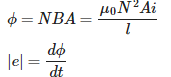QUESTION: 25

If two coils of inductances L1 and L2 are linked such that their mutual inductance is M, then

Solution:

M=k√L1L2

here k is cofficient of coupling. Its maximum value is 1 for tight coupling.

QUESTION: 26

Eddy Currents are

Solution:

Explanation:Eddy currents  (also called Foucault currents) are loops of electrical current induced within conductors by a changing magnetic field in the conductor, due to Faraday's law of induction. Eddy currents flow in closed loops within conductors, in planes perpendicular to the magnetic field. By lenz's law an eddy current creates a magnetic field that opposes a change in the magnetic field that created it, and thus eddy currents react back on the source of the magnetic field.

QUESTION: 27

Polarity of the capacitor in the situation described by the adjacent figure is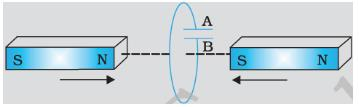Solution:

Explanation:A will become positive with respect to B because current  indused is in clockwise direction

QUESTION: 28

A 1.0 m long metallic rod is rotated with an angular frequency of 400 rad s−1 about an axis normal to the rod passing through its one end. The other end of the rod is in contact with a circular metallic ring. A constant and uniform magnetic field of 0.5 T parallel to the axis exists everywhere. Emf developed between the centre and the ring is

Solution: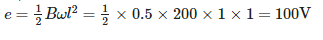QUESTION: 29

On a cylindrical rod two coils are wound one above the other. What is the coefficient of mutual inductance if the inductance of each coil os 0.1H?

Solution:

As one coil is wound over the other so that coupling is tight i.e. k=1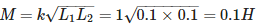QUESTION: 30

Two ends of a horizontal conducting rod of length l are joined to a voltmeter. The whole arrangement moves with a horizontal velocity v, the direction of motion being perpendicular to the rod. Vertical component of the earth's magnetic field is B. The voltmeter reads

Solution: